Command Reference : Matrix Language Reference

 @duplicinv
Inverse duplication matrix.
Syntax: @duplicinv(n)
n: integer
Return: matrix
The inverse of the duplication matrix transforms the vectorization of a sym matrix to the half vectorization of the matrix.
Returns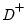, the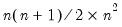Moore-Penrose inverse of the duplication matrix where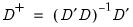for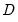, the duplication matrix satisfying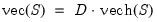for an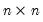symmetric matrix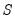, so that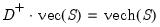Examples
sym s1 = @inner(@mnrnd(10, 3))
matrix idup1 = @duplicinv(s1)
generates a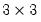symmetric matrix, and computes the inverse duplication matrix.
Similarly, the commands
sym s2 = @unvech(@mnrnd(15))
vector diff = @duplicinv(s2.@cols) * @vec(s2) - @vech(s2)
demonstrates the properties of the inverse duplication matrix since DIFF equals zero.
Cross-references